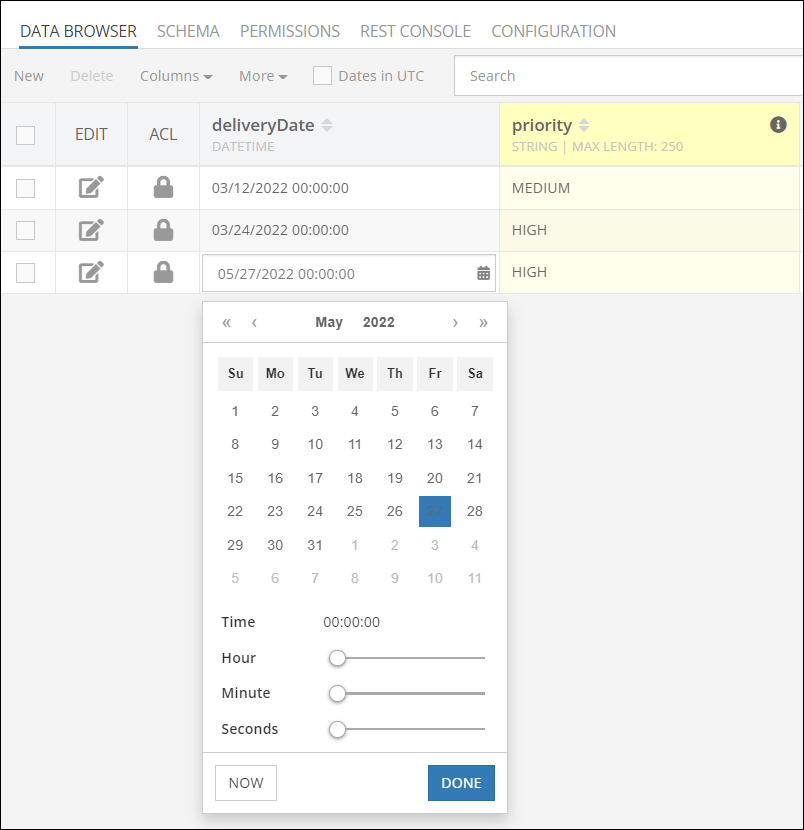# Generated Columns¶

Using this feature, you can declare a new type of column for which the database calculates the values based on a specified expression. These new columns function much like formulas in your favorite spreadsheet application. When you add a generated column, you provide the formula and the database automatically calculates the results based on the data in your database. We call these types of columns “Generated” because the value for these columns is dynamically generated. When the data in the database changes, the value in a generated column changes with it. There is a very rich “expression language” that supports a variety of functions to work on data. Below is a brief video overview of the feature:

## Example¶

Suppose a table has `INT` columns called `op1` and `op2`. The columns can contain numeric values: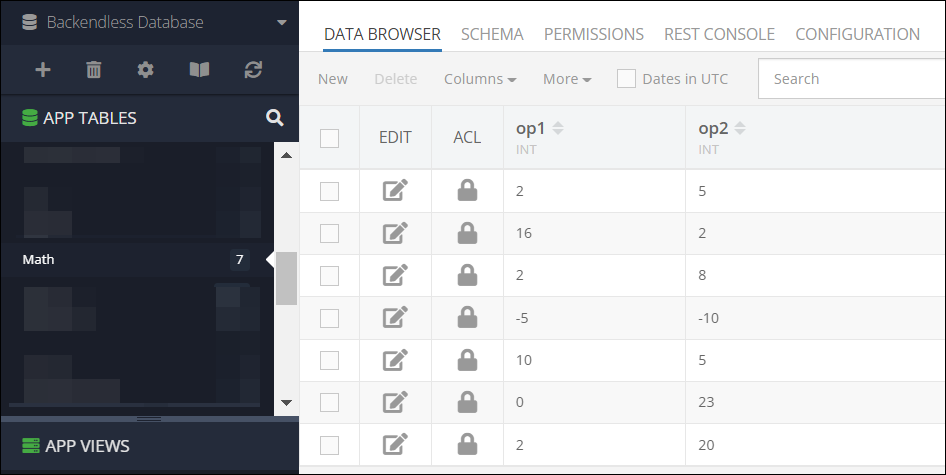The following are some examples of the generated columns:

• A column that calculates the sum of `op1` and `op2` can be declared like this: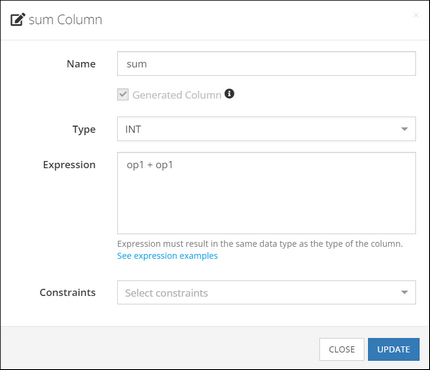• A column that calculates the product of `op1` and `op2`: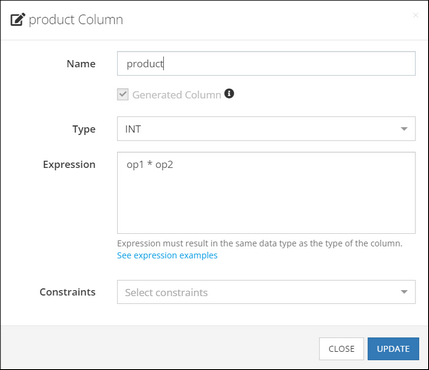• A column that figures out if the `op1` value is negative, zero or positive: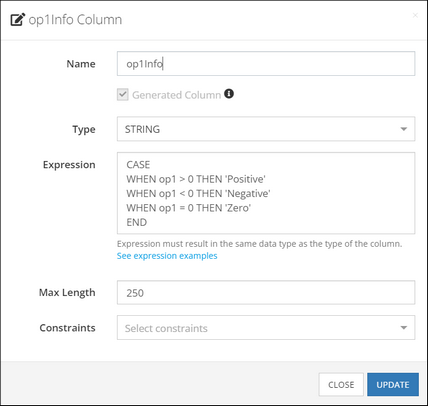Based on the examples above, here’s what we have when the database gets real values for `op1` and `op2`: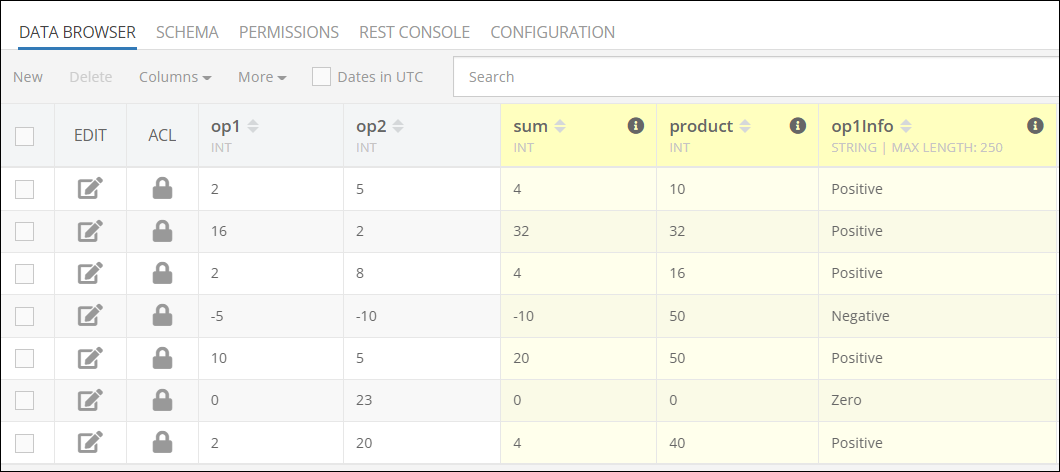To create a generated column for a table, select the table in Backendless Console and switch to the `SCHEMA` tab. Start creating a new column as you normally would and select the `Generated Column` checkbox. Upon the checkbox selection, the user interfaces will automatically change to accommodate generated column parameters: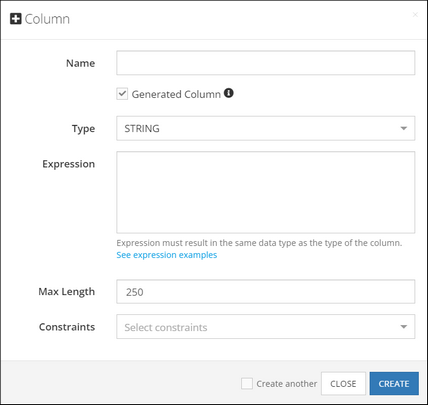## Supported Functions¶

The expression of a generated column can use any of the supported database functions. Additionally, the following functions are also supported:

### Math Functions¶

• `ABS( value or columnName )` - returns absolute value.
• `MOD( value1 or columnName1, value2 or columnName2 )` – Modulo operation. Returns the remainder of the first argument divided by the second argument.
• `FLOOR( value or columnName )` - returns the largest integer value not greater than value.
• `RAND()` - returns a random floating-point value in the range between 0 and 1.0.To obtain a random integer `R` in the range `i <= R < j`, use the expression:
`FLOOR(i + RAND() * (j − i))`
For example, to obtain a random integer in the range `7 <= R < 12`, use the following expression:
`FLOOR(7 + (RAND() * 5))`

The `value or columnName` argument can be:
- a scalar value
- name of a column in the same table
- another function whose result will be used as the input

### String Functions¶

Suppose there is a table with two columns of the `STRING` type. The column names are `firstName` and `lastName`: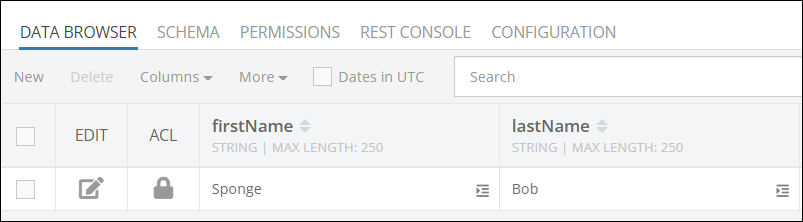Here are some examples of what you can do with generated columns and these string values:

• `CONCAT( value1, value2, …, valueN )` - concatenate values.
Example: `CONCAT( firstName, ' ', lastName )` produces `"Spong Bob"`
• `CONCAT_WS( separator, value1 value2, …, valueN )` - concatenate values with separator.
Example: `CONCAT_WS( ', ', firstName, lastName )` produces `"Sponge, Bob"`
• `TO_BASE64( value )` - encodes value using the base-64 encoding.
Example: `TO_BASE64( firstName )` produces `U3Bvbmdl`
• `FROM_BASE64( value )` - decodes a base-64 encoded value.
Example: `FROM_BASE64( TO_BASE64( firstName ) )` produces `"Sponge"`
• `LENGTH( value )` - returns the length of value measured in bytes.
Example: `LENGTH( firstName )` produces `6`
• `LOWER( value )` - returns value in lower case.
Example: `LOWER( firstName )` produces `"sponge"`
• `UPPER( value )` - returns value in upper case.
Example: `UPPER( CONCAT( firstName, ' ', lastName ) )` produces "SPONGE BOB"

### Conditional Statements¶

It is possible to create a generated column with an expression that includes some conditional logic. Consider the following example, it is a table called `Order`.  The table has a column called `deliveryDate` of the `DATETIME` type. Suppose we need to determine the priority of delivery depending on the day of the week of the delivery date. If the delivery date is between Monday through Friday, the priority is `"HIGH"` and if it is on the weekend (Saturday or Sunday), the priority is `"MEDIUM"`. Here’s what the expression for the generated column could look like:

``````CASE
WHEN DAYOFWEEK( deliveryDate ) IN (2,3,4,5,6)
THEN 'HIGH'
WHEN DAYOFWEEK( deliveryDate ) IN (1,7)
THEN 'MEDIUM'
END
``````

When creating a generated column, the screen will look as shown below: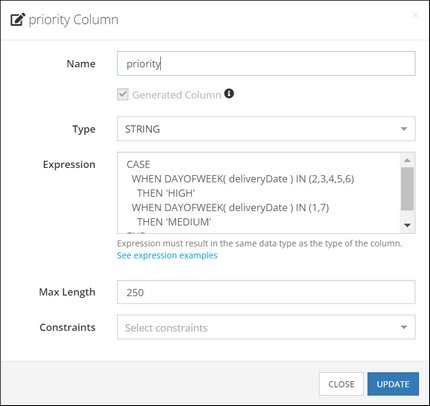Once in place, the database performs the calculation automatically: